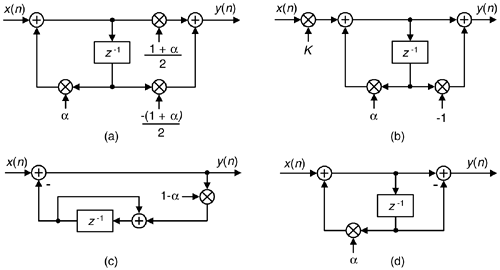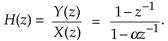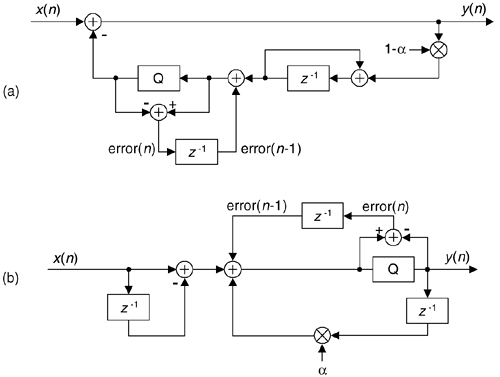# DC REMOVAL

When we digitize analog signals using an analog-to-digital (A/D) converter, the converter's output typically contains some small DC bias: that is, the average of the digitized time samples is not zero. That DC bias may have come from the original analog signal or from imperfections within the A/D converter. Another source of DC bias contamination in DSP is when we truncate a discrete sequence from a B-bit representation to word widths less than B bits. Whatever the source, unwanted DC bias on a signal can cause problems. When we're performing spectrum analysis, any DC bias on the signal shows up in the frequency domain as energy at zero Hz, the X(0) spectral sample. For an N-point FFT the X(0) spectral value is proportional to N and becomes inconveniently large for large-sized FFTs. When we plot our spectral magnitudes, the plotting software will accommodate any large X(0) value and squash down the remainder of the spectrum in which we are more interested.

A non-zero DC bias level in audio signals is particularly troublesome because concatenating two audio signals, or switching between two audio signals, results in unpleasant audible clicks. In modern digital communications systems, a DC bias on quadrature signals degrades system performance and increases bit error rates. With that said, it's clear that methods for DC removal are of interest to many DSP practitioners.

13.23.1 Block-Data DC Removal

If you're processing in non-real-time, and the signal data is acquired in blocks (fixed-length sequences) of block length N, DC removal is straightforward. We merely compute the average of our N time samples, and subtract that average value from each original sample to yield a new time sequence whose DC bias will be extremely small.

This scheme, although very effective, is not compatible with continuous-throughput (real-time) systems. For real-time systems we're forced to use filters for DC removal.

13.23.2 Real-Time DC Removal

The author has encountered three proposed filters for DC removal[55–57]; their structures are shown in Figure 13-62(a), (b), and (c).

Figure 13-62. Filters used for DC bias removal.Ignoring the constant gains of those DC-removal filters, all three filters have identical performance with the general DC-removal filter structure in Figure 13-62(d) having a z-domain transfer function of(It's not immediately obvious that the filters in Figure 13-62(c) and (d) are equivalent. You can verify that equivalency by writing the time-domain difference equations relating the various nodes in the feedback path of Figure 13-62(c)'s filter. Next, convert those equations to z-transform expressions and solve for Y(z)/X(z) to yield Eq. (13-118)).

Because the DC-removal filters can be modeled with the general DC-removal filter in Figure 13-62(d), we provide the general filter's frequency magnitude and phase responses in Figure 13-63(a) and (b) for a = 0.95. The filter's pole/zero locations are given in Figure 13-63(c), where a zero resides at z = 1 providing infinite attenuation at DC (zero Hz) and a pole at z = a making the magnitude notch at DC very sharp. The closer a is to unity, the narrower the frequency magnitude notch centered at zero Hz. Figure 13-63(d) shows the general filter's unit-sample impulse response.

Figure 13-63. DC-removal filter, a = 0.95: (a) magnitude response; (b) phase response; (c) pole/zero locations; (d) impulse response.Figure 13-64 shows the time-domain input/output performance of the general DC-removal filter (with a = 0.95) when its input is a sinusoid suddenly contaminated with a DC bias of 2 beginning at the 100th time sample and disappearing at the 200th sample. The DC-removal filter works well.

Figure 13-64. DC-removal filter performance: (a) filter input with sudden DC bias; (b) filter output.13.23.3 Real-Time DC Removal with Quantization

Because the general DC-removal filter has feedback the y(n) output samples may require wider binary word widths than those used for the x(n) input samples. This could result in overflows in fixed-point binary implementations. The scaling factors of (1+a)/2 and K, in Figure 13-62(a) and (b), are less than one to minimize the chance of y(n) binary overflow.

In fixed-point hardware the y(n) samples are often truncated to the same word width as the input x(n). This quantization (by means of truncation) will induce a negative DC bias onto the quantized output samples, degrading our desired DC removal. When we truncate a binary sample value, by discarding some of its least significant bits, we induce a negative error in the truncated sample. Fortunately, that error value is available for us to add to the next unquantized signal sample, increasing its positive DC bias. When that next sample is truncated, the positive error we've added minimizes the negative error induced by truncation of the next sample.

Figure 13-65(a) shows the addition of a quantizing sigma-delta modulator to the feedback path of the DC-removal filter given in Figure 13-62(c). The positive error induced by truncation quantization (the Q block) is delayed by one sample time and fed back to the quantizer input. Because the modulator has a noise shaping property where quantization error noise is shifted up in frequency, away from zero Hz (DC), the overall DC bias at the output of the filter is minimized.

Figure 13-65. Two DC-removal filters using fixed-point quantization to avoid data overflow.An equivalent quantization noise shaping process can be applied to a Direct Form I version of the Figure 13-62(d) general DC-removal filter as shown in Figure 13-65(b). Again, the positive quantization error is delayed by one sample time and added to the quantizer input[58–60]. To reiterate, the DC-removal filters in Figure 13-65 are used to avoid binary data overflow, by means of quantization, without the use of scaling multipliers.

URL http://proquest.safaribooksonline.com/0131089897/ch13lev1sec23AmazonPrev don't be afraid of buying books Next